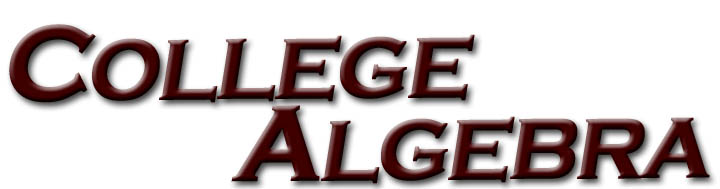# College AlgebraIf you need help in college algebra, you have come to the right place.  Note that you do not have to be a student at WTAMU to use any of these online tutorials.  They were created as a service to anyone who needs help in these areas of math.

If this is your first time using this College Algebra Online Tutorial, please read the Guide to the WTAMU College Algebra Online Tutorial Website to learn how our tutorials are set up and the disclaimer.  Come back to this page to make your tutorial selection.

## Please click on the name of the tutorial of your choice:

Tutorials 2 - 13 cover the prerequisites to College Algebra.  Tutorials 14 on cover the College Algebra concepts.

Tutorial 1:  How to Succeed in a Math Class

Tutorial 2:  Integer Exponents

Tutorial 3:  Scientific Notation

Tutorial 4:  Radicals

Tutorial 5:  Rational Exponents

Tutorial 6:  Polynomials

Tutorial 7:  Factoring Polynomials

Tutorial 8:  Simplifying Rational Expressions

Tutorial 10:  Adding and Subtracting Rational Expressions

Tutorial 11:  Complex Rational Expressions

Tutorial 12:  Complex Numbers

Tutorial 13:  Practice Test on Tutorials 2 - 12

Tutorial 14:  Linear Equation in One Variable

Tutorial 15:  Equations with Rational Expressions

Tutorial 16:  Formulas and Applications

Tutorial 17:  Quadratic Equations

Tutorial 18:  Solving Polynomial Equations by Factoring

Tutorial 20:  Equations that are Quadratic in Form

Tutorial 21:  Absolute Value Equations

Tutorial 22:  Linear Inequalities

Tutorial 23A:  Quadratic Inequalities

Tutorial 23B:  Rational Inequalities

Tutorial 24:  Practice Test on Tutorials 14 - 23

Tutorial 25:  Slope of a Line

Tutorial 26:  Equations of Lines

Tutorial 27:  Graphing Lines

Tutorial 28:  Parallel and Perpendicular Lines

Tutorial 29:  Circles

Tutorial 30:  Introduction to Functions

Tutorial 30B:  Operations with Functions

Tutorial 31:  Graphs of Functions, Part I
(Graphing functions by plotting points)

Tutorial 32:  Graphs of Functions, Part II
(Domain/Range, Vertical Line Test,  Increasing/Decreasing/Constant Functions,  Even/Odd Functions, and Greatest Integer Function)

Tutorial 32B: Inverse Functions

Tutorial 33:  Practice Test on Tutorials 25 - 32

Tutorial 34:  Graphs of Quadratic Functions

Tutorial 35:  Graphs of Polynomial Functions

Tutorial 36:   Long Division

Tutorial 38:   Zeros of Polynomial Functions, Part I
(Rational Zero Theorem and Descartes's Rule of Signs)

Tutorial 39:   Zeros of Polynomial Functions, Part II

Tutorial 40:   Graphs of Rational Functions

Tutorial 41:   Practice Test on Tutorials 34 - 40

Tutorial 42:   Exponential Functions

Tutorial 43:   Logarithmic Functions

Tutorial 44:   Logarithmic Properties

Tutorial 45:   Exponential Equations

Tutorial 46:   Logarithmic Equations

Tutorial 48:   Practice Test on Tutorials 42 - 47

Tutorial 53:    Practice Test on Tutorials 49 - 52

Tutorial 54A:   Sequences

Tutorial 54B:   Series

Tutorial 54C:   Arithmetic Sequences and Series

Tutorial 54D:   Geometric Sequences and Series

Tutorial 54:     The Binomial Theorem

Tutorial 55:     The Fundamental Counting Principle

Tutorial 56:     Permutations

Tutorial 57:     Combinations

Tutorial 58:     Probability

Tutorial 59:     Practice Test on Tutorials 54A - 58[Copyright]  [Fair Use]  [Intellectual Property]  [Resource Guide]

 If you have any comments about this website email Kim Seward at kseward@mail.wtamu.edu This site is brought to you by West Texas A&M University (WTAMU). It was created by Kim Seward with the assistance of Jennifer Puckett. It is currently being maintained by Kim Seward. Disclaimer: WTAMU and Kim Seward are not responsible for how a student does on any test or any class for any reason including not being able to access the website due to any technology problems. We cannot guarantee that you will pass your math class after you go through this website. However, it will definitely help you to better understand the topics covered. Throughout this website, we link to various outside sources. WTAMU and Kim Seward do not have any ownership to any of these outside websites and cannot give you permission to make any kind of copies of anything found at any of these websites that we link to. It is purely for you to link to for information or fun as you go through the study session. Each of these websites have a copy right clause that you need to read carefully if you are wanting to do anything other than go to the website and read it. We discourage any illegal use of the webpages found at these sites. All contents copyright (C) 2001 - 2011, WTAMU, Kim Seward. All rights reserved. Last revised on April 6, 2011 by Kim Seward.